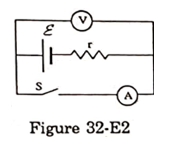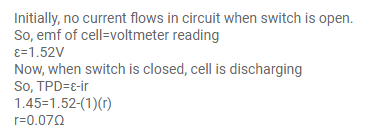# Figure (32-E2) shows an arrangement to measure theQuestion:

Figure (32-E2) shows an arrangement to measure the emf $\varepsilon$ and internal resistance r of battery. The voltmeter has a very high resistance and ammeter also has some resistance. The voltmeter reads $1.52 \mathrm{~V}$ when the switch $\mathrm{S}$ is open. When the switch is closed the voltmeter reading drops to $1.45 \mathrm{~V}$ and ammeter reads $1.0 \mathrm{~A}$. find the emf and internal resistance of the battery.Solution: